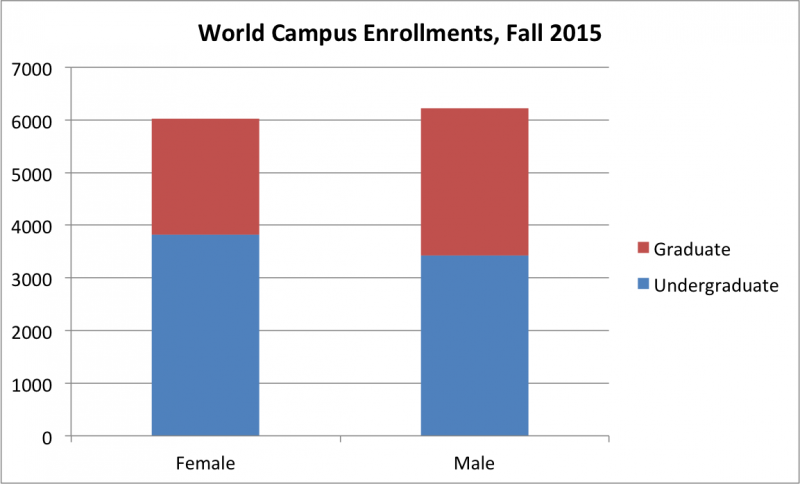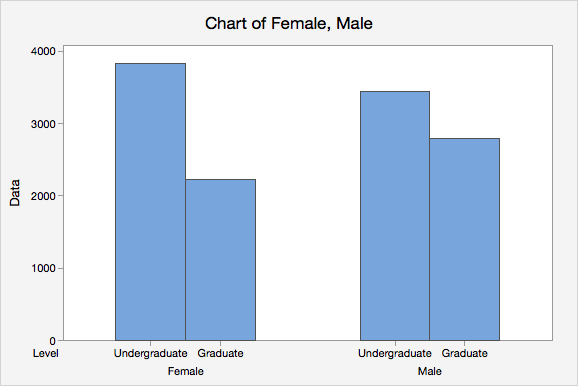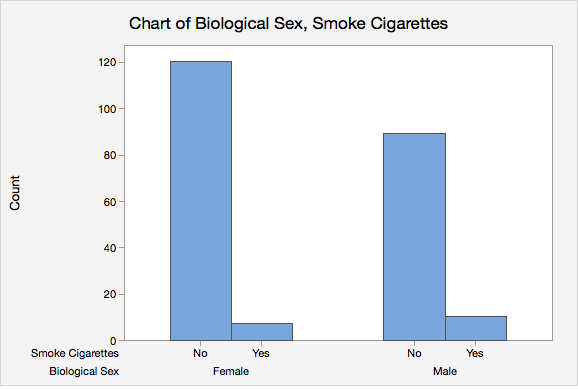# 2.1.2 - Two Categorical Variables

2.1.2 - Two Categorical Variables

Data concerning two categorical variables may be communicated using a two-way table, also known as a contingency table. Data concerning two categorical variables can visualized using a segmented bar chart or a clustered bar chart. A clustered bar chart is also known as a side-by-side bar chart.

Two-Way Table
A display of counts for two categorical variables in which the rows represent one variable and the columns represent a second variable. Also known as a contingency table.

## Example: World Campus Enrollments by Sex

We will use the two-way table below of Penn State World Campus enrollments by biological sex and level to walk through a few examples of how to read a two-way table. These data are from the Penn State Factbook and from the Fall of 2015.

Female Male Total
Total 6027 6215 12242

What proportion of the population of World Campus students is undergraduate?

$p=\frac{7242}{12242}=0.596$

#### Proportion of Females who are Undergraduates

What proportion of females in this population are undergraduate students?

$p=\frac{3814}{6027}=0.633$

Later in this lesson, you will learn that this is known as a conditional probability.

#### Proportion of Undergraduates who are Female

What proportion of undergraduate students in this population are female?

$p=\frac{3814}{7242}=0.527$

Segmented Bar Chart

Also known as a stacked bar chart, one categorical variable is represented on the x-axis while the second categorical variable is denoted within the bars. Minitab Express will not construct a stacked bar chart, but other softwares will. The segmented bar chart below was constructed using Excel.Clustered Bar Chart

Each bar represents one combination of the two categorical variables (i.e., one cell in a contingency table). This is also known as a side-by-side bar chart.# 2.1.2.1 - Minitab Express: Two-Way Table

2.1.2.1 - Minitab Express: Two-Way Table

This dataset consists of STAT 200 students' responses to survey. We can construct a two-way table showing the relationship between Smoke Cigarettes (row variable) and Biological Sex (column variable) using Minitab Express.

## MinitabExpress – Two-Way Table

To create a two-way table in Minitab Express:

1. Open the data set:
2. On a PC: Select STATISTICS > Cross Tabulation and Chi-square
On a Mac: Select Statistics > Tables > Cross Tabulation and Chi-Square
3. Select Raw data (categorical variable) from the drop down menu
4. Double click the variable Smoke Cigarettes in the box on the left to insert the variable into the Rows box
5. Double click the variable Biological Sex in the box on the left to insert the variable into the Columns box
6. Click OK

This should result in the two-way table below:

Tabulated Statistics: Smoke Cigarettes, Biological Sex
Female Male All 120 89 209 7 10 17 127 99 226 Cell Contents: Count
Video Walkthrough

Select your operating system below to see a step-by-step guide for this example.

# 2.1.2.2 - Minitab Express: Clustered Bar Chart

2.1.2.2 - Minitab Express: Clustered Bar Chart

We are going to use the the Class Survey data set in this example again:

## MinitabExpress – Clustered Bar Chart

To create a clustered bar chart in Minitab Express:

1. Open the data set:
2. On a PC or Mac: Select Graphs > Bar Chart
3. In this example we have a datafile with the responses from each case so for Bars represent select Counts of unique values in a categorical variable
4. Select Clustered
5. Double click the variables Biological sex and Smoke Cigarettes in the box on the left to insert the variable into the Categorical variables box
6. Click OK

This should result in the clustered bar chart below:Video Walkthrough

Select your operating system below to see a step-by-step guide for this example.

Note: The order in which the variables are entered into the Categorical variables box in Minitab Express determines how the bars will be clustered. For example, if we entered Smoke Cigarettes and then Gender, the result would be the following clustered bar chart:Link ↥ Has Tooltip/Popover Toggleable Visibility